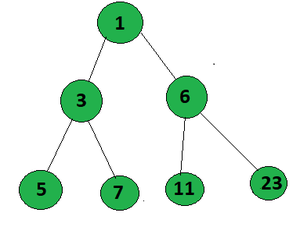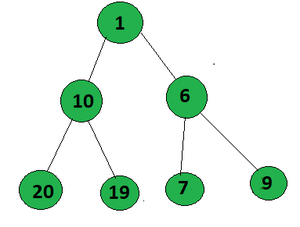Related Articles

# Count of root to leaf paths in a Binary Tree that form an AP

• Last Updated : 23 Aug, 2021

Given a Binary Tree, the task is to count all paths from root to leaf which forms an Arithmetic Progression.

Examples:

Input:Output:
Explanation:
The paths that form an AP in the given tree from root to leaf are:

• 1->3->5 (A.P. with common difference 2)
• 1->6->11 (A.P. with common difference 5)

Input:Output:
Explanation:
The path that form an AP in the given tree from root to leaf is 1->10->19 (A.P. with difference 9)

Approach: The problem can be solved using the Preorder Traversal. Follow the steps below to solve the problem:

• Perform Preorder Traversal on the given binary tree.
• Initialize an array arr[] to store the path.
• Initialize count = 0, to store the count of paths which forms an A.P.
• After reaching the leaf node, check if the current elements in the array(i.e. the node values from root to leaf path) forms an A.P..
• If so, increment the count
• After the complete traversal of the tree, print the count.

Below is the implementation of above approach:

## C++

 `// C++ implementation to count``// the path which forms an A.P.``#include ``using` `namespace` `std;` `int` `count = 0;` `// Node structure``struct` `Node {``    ``int` `val;``    ``// left and right child of the node``    ``Node *left, *right;``    ``// initialization constructor``    ``Node(``int` `x)``    ``{``        ``val = x;``        ``left = NULL;``        ``right = NULL;``    ``}``};` `// Function to check if path``// format A.P. or not``bool` `check(vector<``int``> arr)``{` `    ``if` `(arr.size() == 1)``        ``return` `true``;` `    ``// if size of arr is greater than 2``    ``int` `d = arr - arr;` `    ``for` `(``int` `i = 2; i < arr.size(); i++) {``        ``if` `(arr[i] - arr[i - 1] != d)``            ``return` `false``;``    ``}` `    ``return` `true``;``}` `// Function to find the maximum``// setbits sum from root to leaf``int` `countAP(Node* root, vector<``int``> arr)``{``    ``if` `(!root)``        ``return` `0;` `    ``arr.push_back(root->val);` `    ``// If the node is a leaf node``    ``if` `(root->left == NULL``        ``&& root->right == NULL) {``        ``if` `(check(arr))``            ``return` `1;``        ``return` `0;``    ``}` `    ``// Traverse left subtree``    ``int` `x = countAP(root->left, arr);` `    ``// Traverse the right subtree``    ``int` `y = countAP(root->right, arr);` `    ``return` `x + y;``}` `// Driver Code``int` `main()``{``    ``Node* root = ``new` `Node(1);``    ``root->left = ``new` `Node(3);``    ``root->right = ``new` `Node(6);``    ``root->left->left = ``new` `Node(5);``    ``root->left->right = ``new` `Node(7);``    ``root->right->left = ``new` `Node(11);``    ``root->right->right = ``new` `Node(23);` `    ``cout << countAP(root, {});` `    ``return` `0;``}`

## Java

 `// Java implementation to count``// the path which forms an A.P.``import` `java.util.*;` `class` `GFG{` `int` `count = ``0``;` `// Node structure``static` `class` `Node``{``    ``int` `val;``    ` `    ``// left and right child of the node``    ``Node left, right;``    ` `    ``// Initialization constructor``    ``Node(``int` `x)``    ``{``        ``val = x;``        ``left = ``null``;``        ``right = ``null``;``    ``}``};` `// Function to check if path``// format A.P. or not``static` `boolean` `check(Vector arr)``{``    ``if` `(arr.size() == ``1``)``        ``return` `true``;` `    ``// If size of arr is greater than 2``    ``int` `d = arr.get(``1``) - arr.get(``0``);` `    ``for``(``int` `i = ``2``; i < arr.size(); i++)``    ``{``        ``if` `(arr.get(i) - arr.get(i - ``1``) != d)``            ``return` `false``;``    ``}``    ``return` `true``;``}` `// Function to find the maximum``// setbits sum from root to leaf``static` `int` `countAP(Node root,``                   ``Vector arr)``{``    ``if` `(root == ``null``)``        ``return` `0``;` `    ``arr.add(root.val);` `    ``// If the node is a leaf node``    ``if` `(root.left == ``null` `&&``        ``root.right == ``null``)``    ``{``        ``if` `(check(arr))``            ``return` `1``;``        ``return` `0``;``    ``}` `    ``// Traverse left subtree``    ``int` `x = countAP(root.left, arr);` `    ``// Traverse the right subtree``    ``int` `y = countAP(root.right, arr);` `    ``return` `x + y;``}` `// Driver Code``public` `static` `void` `main(String[] args)``{``    ``Node root = ``new` `Node(``1``);``    ``root.left = ``new` `Node(``3``);``    ``root.right = ``new` `Node(``6``);``    ``root.left.left = ``new` `Node(``5``);``    ``root.left.right = ``new` `Node(``7``);``    ``root.right.left = ``new` `Node(``11``);``    ``root.right.right = ``new` `Node(``23``);` `    ``System.out.print(countAP(root, ``new` `Vector()));``}``}` `// This code is contributed by gauravrajput1`

## Python3

 `# Python3 implementation to count``# the path which forms an A.P.` `# Node structure``class` `Node:``    ``def` `__init__(``self``, x):``        ` `        ``self``.val ``=` `x``        ``self``.left ``=` `None``        ``self``.right ``=` `None``        ` `# Function to check if path``# format A.P. or not``def` `check(arr):``    ` `    ``if` `len``(arr) ``=``=` `1``:``        ``return` `True``    ` `    ``# If size of arr is greater than 2``    ``d ``=` `arr[``1``] ``-` `arr[``0``]``    ` `    ``for` `i ``in` `range``(``2``, ``len``(arr)):``        ``if` `arr[i] ``-` `arr[i ``-` `1``] !``=` `d:``            ``return` `False``            ` `    ``return` `True` `# Function to find the maximum``# setbits sum from root to leaf``def` `countAP(root, arr):``    ` `    ``if` `not` `root:``        ``return` `0``    ` `    ``arr.append(root.val)``    ` `    ``# If the node is a leaf node``    ``if` `(root.left ``=``=` `None` `and``        ``root.right ``=``=` `None``):``        ``if` `check(arr):``            ``return` `1``        ``return` `0``    ` `    ``# Traverse the left subtree``    ``x ``=` `countAP(root.left, arr)``    ` `    ``# Traverse the right subtree``    ``y ``=` `countAP(root.right, arr)``    ` `    ``return` `x ``+` `y` `# Driver code``root ``=` `Node(``1``)``root.left ``=` `Node(``3``)``root.right ``=` `Node(``6``)``root.left.left ``=` `Node(``5``)``root.left.right ``=` `Node(``7``)``root.right.left ``=` `Node(``11``)``root.right.right ``=` `Node(``23``)` `print``(countAP(root, []))` `# This code is contributed by stutipathak31jan`

## C#

 `// C# implementation to count``// the path which forms an A.P.``using` `System;``using` `System.Collections.Generic;` `class` `GFG{` `//int count = 0;` `// Node structure``class` `Node``{``    ``public` `int` `val;``    ` `    ``// left and right child of the node``    ``public` `Node left, right;``    ` `    ``// Initialization constructor``    ``public` `Node(``int` `x)``    ``{``        ``val = x;``        ``left = ``null``;``        ``right = ``null``;``    ``}``};` `// Function to check if path``// format A.P. or not``static` `bool` `check(List<``int``> arr)``{``    ``if` `(arr.Count == 1)``        ``return` `true``;` `    ``// If size of arr is greater than 2``    ``int` `d = arr - arr;` `    ``for``(``int` `i = 2; i < arr.Count; i++)``    ``{``        ``if` `(arr[i] - arr[i - 1] != d)``            ``return` `false``;``    ``}``    ``return` `true``;``}` `// Function to find the maximum``// setbits sum from root to leaf``static` `int` `countAP(Node root,``                   ``List<``int``> arr)``{``    ``if` `(root == ``null``)``        ``return` `0;` `    ``arr.Add(root.val);` `    ``// If the node is a leaf node``    ``if` `(root.left == ``null` `&&``       ``root.right == ``null``)``    ``{``        ``if` `(check(arr))``            ``return` `1;``        ``return` `0;``    ``}` `    ``// Traverse left subtree``    ``int` `x = countAP(root.left, arr);` `    ``// Traverse the right subtree``    ``int` `y = countAP(root.right, arr);` `    ``return` `x + y;``}` `// Driver Code``public` `static` `void` `Main(String[] args)``{``    ``Node root = ``new` `Node(1);``    ``root.left = ``new` `Node(3);``    ``root.right = ``new` `Node(6);``    ``root.left.left = ``new` `Node(5);``    ``root.left.right = ``new` `Node(7);``    ``root.right.left = ``new` `Node(11);``    ``root.right.right = ``new` `Node(23);` `    ``Console.Write(countAP(root, ``new` `List<``int``>()));``}``}` `// This code is contributed by amal kumar choubey`

## Javascript

 ``

Output:

`2`

Time Complexity: O(N)
Auxiliary Space: O(h), where h is the height of binary tree.

Attention reader! Don’t stop learning now. Get hold of all the important DSA concepts with the DSA Self Paced Course at a student-friendly price and become industry ready.  To complete your preparation from learning a language to DS Algo and many more,  please refer Complete Interview Preparation Course.

In case you wish to attend live classes with experts, please refer DSA Live Classes for Working Professionals and Competitive Programming Live for Students.

My Personal Notes arrow_drop_up